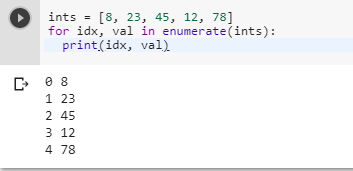# Accessing the index in 'for' loops?

1 view
in Python
edited

How do I access the index itself for a list like the following?

ints = [8, 23, 45, 12, 78]

for i in ints:

print('item #{} = {}'.format(???, i))

I want to get this output:

item #1 = 8

item #2 = 23

item #3 = 45

item #4 = 12

item #5 = 78

When I loop through it using a for loop, how do I access the loop index, from 1 to 5 in this case?

by (107k points)

If you want to access the index in 'for' loop then you can use the built-in Python function enumerate(), this function is available in both Python 2 and 3 below is the piece of code that uses enumerate() function:-

ints = [8, 23, 45, 12, 78]

for idx, val in enumerate(ints):

print(idx, val)